# Raspberries

Dano had 20 raspberries. John had 90% more raspberries than Dano. Determine how many raspberries have Dano and Juraj together.

Correct result:

x =  58

#### Solution: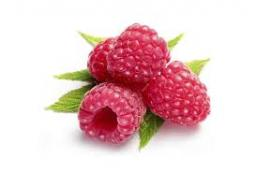We would be pleased if you find an error in the word problem, spelling mistakes, or inaccuracies and send it to us. Thank you!Tips to related online calculators

## Next similar math problems:

• Geography testsOn three 150-point geography tests, you earned grades of 88%, 94%, and 90%. The final test is worth 250 points. What percent do you need on the final to earn 93% of the total points on all tests?
• Sum of three numbersThe sum of three numbers from which second number is 20% smaller than the first number and the third number is 25% smaller than the second number is 96. Determine this numbers.
• Price increase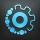A 20 percent price increase meant a 90-crown raise. How many cost a product after?
• Percent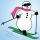From 99 children who participated in the ski course were 23 excellent skiers, 13 good and 20 average and the rest were beginners. Calculate this data in percentages.
• Percent calculationCalculate 51% if 99% is 79.
• Acid solutionBy adding 250 grams of a 96% solution of sulfuric acid to its 3% solution its initial concentration was changed to 25%. How many grams of 3% of the acid were used for dilution?
• Price saleoffShoes standing y euros. At first they were discounted by 12% and then 50% of the new amount. After this double cheapening the cost was exactly 22 euros. Determine the original price of shoes.
• NumberWhat number is 20 % smaller than the number 198?
• Value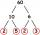Determine the value of the following exspressions: a) (23-25)·(4-5) b) (97-123):(18+8)
• One percent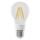One percent of all the lights in the city are LED, the remaining 99% are conventional. Other types are not there. John counted them honestly but he had counted only conventional. After a good dinner he registered numbers and he have notice that from all t
• Unknown number 716% of the unknown number is by 21 less than unknown number itself. Determine the natural unknown number.
• Unknown number 24f we add 20, we get 50% of its triple. What is this unknown number?
• Four numbersThe first number is 50% second, the second number is 40% third, the third number is 20% of the fourth. The sum is 396. What are the numbers?
• Farmer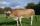The farmer farming 293 ha of arable land and the remaining 20% are meadows and pastures. How much land farmer owns?
• KiteJohn a kite, which is diamond shaped. Its diagonals are 60 cm long and 90 cm long. Calculate: a) the diamond side b) how much paper John needs to make a kite if he needs paper on both sides and needs 5% of the paper for bending.
• Ethnicity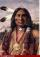Share of ethnicity XY is 26%, which is 1/9 more than in the prewar period. What was the share of that ethnicity in the pre-war period?
• The notebook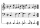After rising by 40%, the notebook cost 10.50 euros. How much did this notebook cost if it increased in price by only 20% instead of 40%?